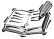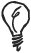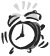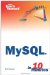# Numeric Functions

A function is a MySQL command used in an SQL statement that takes one or more arguments and returns a value based on the values supplied. Just as with expressions, you can use a function anywhere a fixed value could otherwise be used, or to manipulate the value returned in a database column.## Case Sensitivity

Function names are not case sensitive in MySQL. For example, you can use ROUND(), Round(), or round()these all perform the same function call.## Arguments

To pass a function more than one argument, separate the values in parentheses using a comma. If a function does not require any arguments, the parentheses must still be givenfor instance, RAND().

#### Random Numbers

To generate a random number, use the RAND() function with no arguments. The result is a random decimal number between 0 and 1.

` mysql> SELECT RAND(), RAND(); +------------------+------------------+ | RAND()           | RAND()           | +------------------+------------------+ | 0.96684006784748 | 0.27750918760664 | +------------------+------------------+ 1 row in set (0.00 sec) `

In the previous example, two different calls to RAND() caused two different random numbers to be generated; a different value is returned each time RAND() is called, even within the same query.## Shuffling a Query

You can use the RAND() function in an ORDER BY clause to shuffle the order in which records are returned. Simply use ORDER BY RAND(); the value of RAND() will be different for each row returned by the query, and the results will be sorted randomly according to this value.

An optional argument to RAND() specifies the random number seed. This resets the random number generator to a particular point in its sequence. Whenever the sequence is reset to a known point, the same sequence of random numbers will be generated.## Random Seeds

You should never need to seed the random number generator with an argument to RAND(). Calling the function without an argument will give a number that can be considered random.

#### Rounding Functions

This set of functions enables you to round decimal numbers in a particular way. Each of these functions takes a single decimal number argument and returns an integer result.## Decimals and Integers

In this book, we refer to whole numbers as integers and fractional numbers as decimals. These terms correspond to the MySQL column data types, which you will learn about in Lesson 14, "Creating and Modifying Tables."

The ROUND() function rounds a value up or down to the nearest integer.

` mysql> SELECT ROUND(29.21), ROUND(32.76); +--------------+--------------+ | ROUND(29.21) | ROUND(32.76) | +--------------+--------------+ |           29 |           33 | +--------------+--------------+ 1 row in set (0.00 sec) `

If you supply a second argument, the number will be rounded to that amount of decimal places instead of an integer.

` mysql> SELECT ROUND(29.21, 1), ROUND(32.76, 1); +-----------------+-----------------+ | ROUND(29.21, 1) | ROUND(32.76, 1) | +-----------------+-----------------+ |            29.2 |            32.8 | +-----------------+-----------------+ 1 row in set (0.00 sec) `

The CEILING and FLOOR functions round a decimal number up and down to the nearest integer, respectively.

` mysql> SELECT CEILING(29.21), FLOOR(29.21); +----------------+--------------+ | CEILING(29.21) | FLOOR(29.21) | +----------------+--------------+ |             30 |           29 | +----------------+--------------+ 1 row in set (0.00 sec) `## Rounding Negatives

Rounding a negative number up produces a higherthat is, less negativevalue. Rounding down produces a lower value. For instance, CEILING(-2.6) returns -2, not -3 because -2 is a numerically greater value than -2.6.

The TRUNCATE function takes two arguments, a number and a precision. It does not round the number, but simply removes from it decimal places in excess of the precision specified.

` mysql> SELECT TRUNCATE(12.34567, 3); +-----------------------+ | TRUNCATE(12.34567, 3) | +-----------------------+ |                12.345 | +-----------------------+ 1 row in set (0.00 sec) `

#### Mathematical Functions

This set of functions enables you to perform mathematical operations in a query.

To raise a number to a power, use the POW() function. It takes two arguments: POW(a,b) raises a to the power b.

` mysql> SELECT POW(2,2), POW(2,3), POW(2,4); +----------+----------+----------+ | POW(2,2) | POW(2,3) | POW(2,4) | +----------+----------+----------+ |        4 |        8 |       16 | +----------+----------+----------+ 1 row in set (0.00 sec)  `

To find the square root of a number, use SQRT() with a single argument. The following example also shows how functions can be nested by putting one function call as the argument to another. It calculates the square root of a number after it has just been squared.

` mysql> SELECT SQRT(POW(8,2)); +-----------------+ | SQRT(POW(8, 2)) | +-----------------+ |               8 | +-----------------+ 1 row in set (0.00 sec) `

To find the logarithm of a number, use the LOG() function. With one argument, a natural logarithm (base e) is returned. The opposite of LOG() is EXP()natural log base e is raised to the power given in the argument.

In the following example, the first column shows the value of e. The second column performs a natural logarithm on this value.

` mysql> SELECT EXP(1), LOG(EXP(1)); +----------------+-------------+ | EXP(1)         | LOG(EXP(1)) | +----------------+-------------+ | 2.718281828459 |           1 | +----------------+-------------+ 1 row in set (0.00 sec) `

When called with two arguments, the LOG() function uses the first argument as its base. For instance, LOG(10,num) returns the logarithm of num to base 10.

#### Trigonometry Functions

MySQL supports trigonometry functions to calculate the sine, cosine, and tangent of a value using SIN(), COS(), and TAN(), respectively.

The argument to these functions should be a value in radians. The following examples use PI() to supply arguments to these functions that are based on [pi].

` mysql> SELECT SIN(PI()/2), COS(2*PI()), TAN(PI()/4); +-------------+-------------+-------------+ | SIN(PI()/2) | COS(2*PI()) | TAN(PI()/4) | +-------------+-------------+-------------+ |           1 |           1 |           1 | +-------------+-------------+-------------+ 1 row in set (0.00 sec) `## Inverse Functions

The inverse functions for SIN(), COS(), and TAN() are ASIN(), ACOS(), and ATAN(), respectively. A valid result will be returned only for arguments between 1 and +1.

To convert a value in degrees to radians, use DEGREES(). Likewise, to convert radians to degrees, use RADIANS().

` mysql> SELECT DEGREES(PI()/2), RADIANS(180); +-----------------+-----------------+ | DEGREES(PI()/2) | RADIANS(180)    | +-----------------+-----------------+ |              90 | 3.1415926535898 | +-----------------+-----------------+ 1 row in set (0.00 sec) `Sams Teach Yourself MySQL in 10 Minutes
ISBN: 0672328631
EAN: 2147483647
Year: 2006
Pages: 165
Authors: Chris Newman

Similar book on Amazon

flylib.com © 2008-2017.
If you may any questions please contact us: flylib@qtcs.net